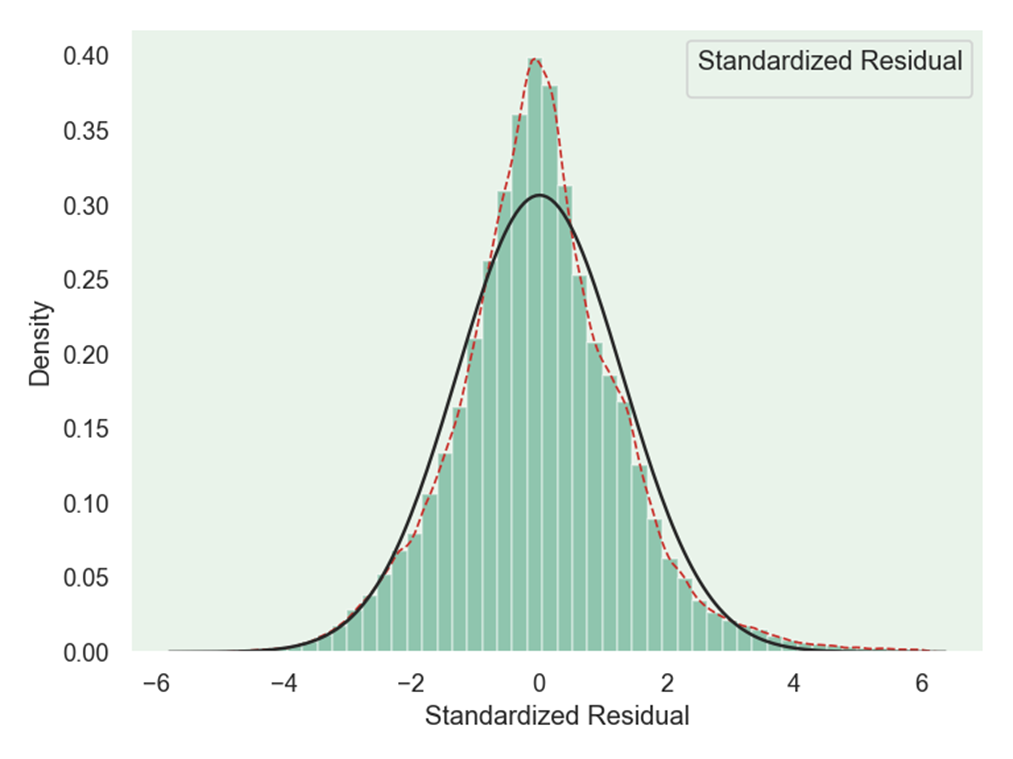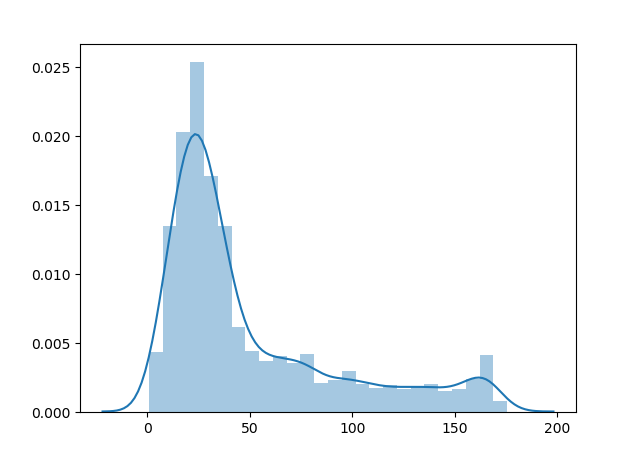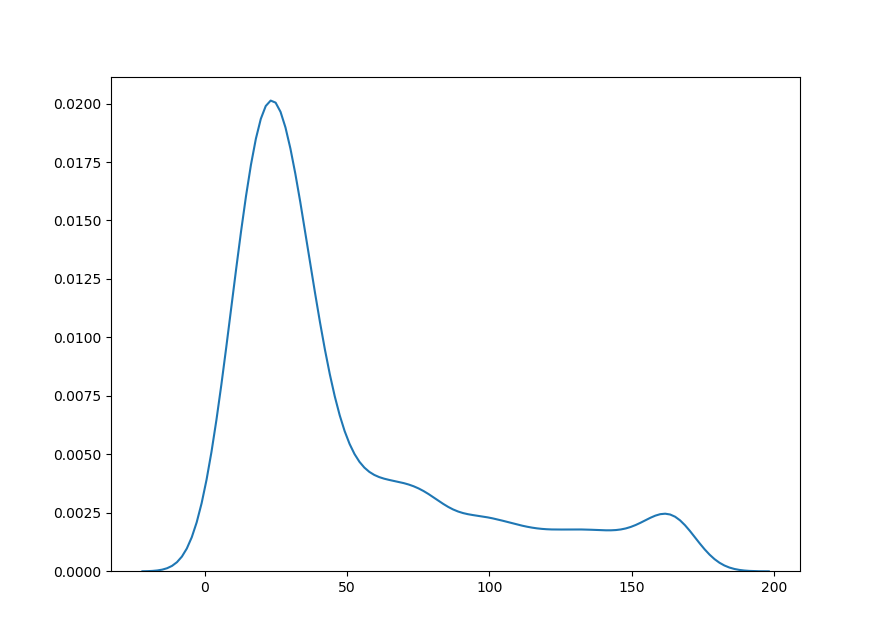• 2021-12-04 09:32:29
for i in sorted(list(set(df['yearmonth']))):
df_sort = df[df['yearmonth'] == i].groupby('cust_id')['y','pred'].sum().sort_values('y',ascending = False)
df_sort['yearmonth'] = i
df_sort['y_cum'] = np.cumsum(df_sort['y'])
df_sort['y_cum_rate'] = df_sort['y_cum']/max(df_1_clear_sort['y_cum'])
df_sort['index'] = list(range(1,df_sort.shape+1))
df_sort['index_cum_rate'] = [i/df_sort.shape for i in list(range(1,df_sort.shape+1))]

plt.figure(figsize=(4,4))
plt.plot(list(df_sort['index_cum_rate']),list(df_sort['y_cum_rate']))
plt.title(i,fontsize=24)
plt.xlabel('index_cum_rate',fontsize=14)
plt.ylabel('y_cum_rate',fontsize=14)
plt.grid(True)
plt.show()

更多相关内容
• matlab作概率密度函数很简单，但是本人学的python，因此想用python作图，从txt文本中读取数据，然后用python概率密度函数图像，
• 当我们想要弄清楚变量的统计特性时，往往想知道它是服从什么分布的，这时候就需要绘制概率分布直方图 ...在seaborn中的distplot函数可以完成概率分布直方图和密度图的绘制 seaborn.distplot(a, bins=None, his.

当我们想要弄清楚变量的统计特性时，往往想知道它是服从什么分布的，这时候就需要绘制概率分布直方图

在python中我们可以使用seaborn库来进行绘制：

Seaborn是一个基于matplotlib的Python数据可视化库。它为绘制有吸引力和信息丰富的统计图形提供了高级界面。

首先需要导入seaborn库：

import seaborn as sns


在seaborn中的distplot函数可以完成概率分布直方图和密度图的绘制

seaborn.distplot(a, bins=None, hist=True, kde=True, rug=False,
fit=None, hist_kws=None, kde_kws=None, rug_kws=None, fit_kws=None,
color=None, vertical=False, norm_hist=False, axlabel=None,
label=None, ax=None)


下面直接给出我绘制时用到的代码：

import seaborn as sns
import matplotlib as mpl
import matplotlib.pyplot as plt
from scipy.stats import norm
from scipy.stats import laplace

txt=[]
data=[]
path = r"E:\rtklib\rtklib-test1\stav.txt"

#mpl.rc("figure", figsize=(9, 5))
with open(path, "r") as f:

for i in range(len(txt)):
data.append(float(txt[i].strip().split(',')))

sns.set_palette("hls")
#sns.set_style("whitegrid")
plt.figure(dpi=120)
sns.set(style='dark')
sns.set_style("dark", {"axes.facecolor": "#e9f3ea"})
g = sns.distplot(data,
hist=True,
kde=True,  # 开启核密度曲线kernel density estimate (KDE)
kde_kws={'linestyle': '--', 'linewidth': '1', 'color': '#c72e29',
# 设置外框线属性
},
fit=norm,
color='#098154',
axlabel='Standardized Residual',  # 设置x轴标题

)

plt.show()


其中，distplot的参数中，kde表示是否绘制核密度曲线；fit是选择拟合的分布，来分析数据究竟是符合什么分布，seaborn中提供了很多分布，可以在这里找到，使用时如上我的代码中导入的那样就可以：连续分布类型
最后的效果图如下：绘制过程中没有加入太多修饰，如果想要好看一点，可以修改一些参数：
其中一个有设置背景 的风格，主要有以下几种：

sns.set_style("whitegrid")
sns.set_style("dark")
sns.set_style("darkgrid")
sns.set_style("white")
sns.set_style("ticks")

展开全文算法
• 我有一个二维，用的是一组“电压值”和它的概率。 在中，红色图形对应一个脉冲长度，绿色图形对应另一个脉冲长度。我用堆栈溢出（Plot normal distribution in 3D）中的一个多元正态分布示例，以同样的方式绘制...

我正在研究一个由“脉冲长度”值和对应于每个脉冲长度的5或6个“电压值”组成的数据集。第一个值是脉冲长度，后面是电压。找到下表。在

15-56伏-47伏-53伏-50伏-50伏

16-49伏-46伏-52伏-47伏-50伏

47伏-50伏-50伏

18-50伏-51伏-48伏-48伏-45伏

19-49伏-51伏-45伏-47伏-52伏

20-45伏-47伏-50伏-47伏-54伏

21-46伏-52伏-52伏-49伏-54伏

22-53伏-51伏-53伏-56伏-52伏

23-52伏-45伏-51伏-56伏-53伏

24-51伏-52伏-54伏-58伏-52伏

25-56伏-53伏-57伏-55伏-53伏

26-53伏-52伏-55伏-52伏

27-54伏-49伏-56伏-54伏

28-52伏-52伏-57伏-56伏-53伏

29-63伏-60伏-54伏-58伏-61伏

30-59伏-70伏-61伏

X轴和Y轴是脉冲长度和电压，Z轴是它的概率分布。我有一个二维图，用的是一组“电压值”和它的概率。在图中，红色图形对应一个脉冲长度，绿色图形对应另一个脉冲长度。我用堆栈溢出(Plot normal distribution in 3D)中的一个多元正态分布示例，以同样的方式绘制3D图。由于我对3D绘图经验不足，我无法在同一个曲面上用不同的Y轴“脉冲长度”值绘制多个曲面图。我尝试的代码如下所示。在import numpy as np

import matplotlib

import matplotlib.pyplot as plt

from matplotlib.mlab import bivariate_normal

from mpl_toolkits.mplot3d import Axes3D

#Parameters to set

mu_x = -48.8

sigma_x = np.sqrt(6.5)

mu_y = 0

sigma_y = np.sqrt(16)

#Create grid and multivariate normal

x = range(-100,0)

y = range(15,30)

X, Y = np.meshgrid(x,y)

Z = bivariate_normal(X,Y,sigma_x,sigma_y,mu_x,mu_y)

#Make a 3D plot

fig = plt.figure()

ax = fig.gca(projection='3d')

ax.plot_surface(X, Y, Z,cmap='Reds',linewidth=0, antialiased=True,

zorder = 0.5)

ax.set_xlabel('Voltage')

ax.set_ylabel('Pulse Length')

ax.set_zlabel('Normal Distribution')

plt.show()

如果有人能帮我做同样的多脉冲长度，我会非常感激。

谢谢您。在

展开全文• kde（核密度估计）是对未知分布的估计，所以可能有超出去值范围的估计（比如下我定义的有效范围是0-180，但kde在<0和>180时仍然有曲线）。只要看自己想要的范围就好了，它在上面的拟合是可以的。 当然，也...

安装：直接pip

kde（核密度估计）是对未知分布的估计，所以可能有超出去值范围的估计（比如下图我定义的有效范围是0-180，但kde在<0和>180时仍然有曲线）。只要看自己想要的范围就好了，它在上面的拟合是可以的。

当然，也可以在distplot中用不同曲线拟合。

import seaborn as sns
from scipy import stats

# 绘制概率分布条形图，此时kde默认为true
sns.distplot(data, rug=False, hist=True)
# 也可以这样画kde
# hist=False表示不要bar
# 这里用gamma分布拟合
sns.distplot(data, hist=False, kde=False, fit=stats.gamma)展开全文• 目录   1、scipy库中各分布对应的方法   2、stats中各分布的常用方法及其功能   3、正态分布的概率密度函数及其图象 ...    3）python绘制t分布和正态分布的概率密度函数对比   6、F分布的概率密度函数
• 主要介绍了python 计算概率密度、累计分布、逆函数的例子,具有很好的参考价值，希望对大家有所帮助。一起跟随小编过来看看吧
• 对于pandas的dataframe，...对于pandas的dataframe，绘制概率密度图方法如下： //pdf是pandas的dataframe, delta_time是其中一列 pdf.delta_time.dropna().plot(kind='kde', xlim=(-50,300)) 以上这篇python绘制直方
• ## 绘制概率密度

千次阅读 2021-02-04 07:12:24
最常见的方法是使用fill_between对置信区间之间的区域进行着色。例如：import numpy as npnp.random.seed(1977)import matplotlib.pyplot as plt# Generate data...x_obs = np.linspace(-2, 2, 20)true_model = [0.2...
• 这篇文章将向您展示如何：使用高斯核估计2个分布的PDF(PDF即Probability Density Function，概率密度函数)使用Matplotlib表示PDF，其中密度图周围带有标记的轮廓线如何提取轮廓线如何在3D(3维空间)中绘制上述高斯核...
• 最初遇到这个问题的时候，找到的大答案是绘制contour，比如： http://stackoverflow.com/questions/24119920/how-to-plot-a-density-map-in-python?rq=1​ 这当然很漂亮，但是这需要有三列数字来标示一个平面；...
• Python绘制概率分布直方 文章目录Python绘制概率分布直方一、代码1、案例一2、案例二二、运行效果1、案例一2、案例二 一、代码 1、案例一 import random import numpy as np import matplotlib.pyplot as plt X...深度学习 机器学习
• ## python画分布、密度等图形

万次阅读 多人点赞 2016-11-29 11:43:47
# 下面的看上去复杂, 不过也很好理解, 从一个样本点生成一个bell-curve # 这样看bell集中的地方就是数据最密集的地方. sns.rugplot(data, color='indianred') xx = np.linspace(-4, 4, 100) # 计算bandwidth ...
• 头大，外行人做个咋这么难，趋势线还没有研究出来怎么加上去，哎 import matplotlib.pyplot as plt from scipy.stats import gaussian_kde from mpl_toolkits.axes_grid1 import make_axes_locatable import ...
• python散点图密度怎么计算关注:218答案:2mip版解决时间 2021-01-25 12:21提问者莪早已看透了誓言2021-01-24 22:16python散点图密度怎么计算最佳答案二级知识专家臸釪樶初2021-01-24 22:53import matplotlib.pyplot ...
• #python 画概率密度图 #-*- coding: utf-8 -*- import matplotlib.pyplot as plt import numpy as np import seaborn as sns # 1）准备数据 lengths = [] with open("D:/length_analysis.tsv","r") as f: for l in...
• mumpy 、random import numpy as np import seaborn as sns import matplotlib.pyplot as plt from scipy import stats np.random.seed(1234) rn1 = np.random.normal(loc = 0, scale = 1, size = 1000) ...
• 本文目标在于利用 PythonPythonPython 快速出符合自己要求的箱图与密度图。 如果你对这篇文章可感兴趣，可以点击「【访客必读 - 指引页】一文囊括主页内所有高质量博客」，查看完整博客分类与对应链接。 箱图 ...
• 关于Python绘制正态分布概率密度分布）以及遇到的问题（label无法显示） 目的：统计神经网络模型的参数分布情况 使用seaborn给我们提供的distplot函数来绘制，即调用sns.distplot()，并传入相关参数即可，这里...数据挖掘 深度学习
• 4 Band 4 0.005679 0.026662 0.014529 0.006505 我想创建一个有8条线的概率密度图，其中4条带代表“红色”，另一条带代表“黑色”，到目前为止，在红色和黑色的roi中，我只有1条带。但是我的代码输出了两个不同的图...
• ## 作图篇：python密度图(Density Plot)

万次阅读 多人点赞 2019-01-21 16:48:58
这种密度图正是可视化连续型随机变量分布的利器，分布曲线上的每一个点都是概率密度，分布曲线下的每一段面积都是特定情况的概率。如下所示，通过将它们按「response」变量分组，我们可以了解 X 轴和 Y 轴之间的关系...
• 四、用Python代码实现常见概率分布 二项分布（伯努利分布） from scipy import stats as st import numpy as np import matplotlib as mpl import matplotlib.pyplot as plt #防止乱码 mpl.rcParams['font.sans-...
• 利用geopandas和已有地理空间数据，结合shp矢量文件绘制感兴趣区域的物种分布和密度图
• 一、关于核密度估计带宽的一些说明进行核密度估计Kernel Density Estimation(KDE)时，带宽bw的选取尤为重要，不同的带宽会对结果造成很大的影响。利用seaborn库中kdeplot()绘制kde曲线时，可以选择scott和silverman...
• Matplotlib在概率密度曲线的基础上绘制积分区域实例matplotlib
• 我想绘制基于概率密度函数的近似值我有一个样本;模拟直方行为的曲线.我可以有我想要的样本.解决方法:如果要绘制分布,并且知道它,请将其定义为函数,并将其绘制为：import numpy as npfrom matplotlib import pyplot......